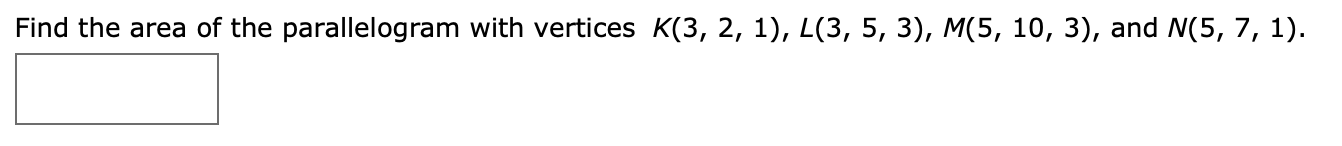# Find the area of the parallelogram with vertices K(3, 2, 1), L(3, 5, 3), M(5, 10, 3), and N(5, 7, 1).

Question
8 viewshelp_outlineImage TranscriptioncloseFind the area of the parallelogram with vertices K(3, 2, 1), L(3, 5, 3), M(5, 10, 3), and N(5, 7, 1). fullscreen
check_circle

Step 1

To find the area of the given parallelogram, use the vector method which is described as.

We know that vector product between two vectors is a vector whose length is the area of the parallelogram that has two vectors as two sides.

So in first step compute the desired vectors which are sides of the parallelogram as.

Step 2

In second step compute the vector pr...

### Want to see the full answer?

See Solution

#### Want to see this answer and more?

Solutions are written by subject experts who are available 24/7. Questions are typically answered within 1 hour.*

See Solution
*Response times may vary by subject and question.
Tagged in
MathCalculus

### Other